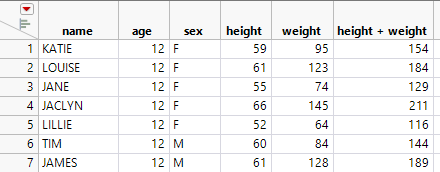Publication date: 05/24/2021

## Formulas in JMP

In spreadsheets, formulas apply to a single cell and can utilize data from any cell in the spreadsheet, including cells on different tabs of the workbook. In data tables, formulas apply to an entire column. A formula can use data from any other column in the data table. Each row in the column will have the same calculation applied to it based on the data in the row.

For example, consider a data table with a simple sum as shown in Figure 2.11. The column height + weight has a formula. The formula adds height and weight by row for all rows in the data table.

Figure 2.11 Data Table with Formula Column